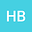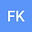Existence of homoclinic solutions for the non-autonomous fractional Hamiltonian systems
••• Hamid Boulares,
• Fathi Khelifi
Hamid Boulares
Université 8 Mai 1945 Guelma Faculté des Mathématiques et de l'Informatique et des Sciences de la Matière
Author ProfileFathi Khelifi
University of Hail College of Sciences
Author Profile## Abstract

In this reseach work, we give a new result to guarantee the existence of homoclinic solutions for the nonperiodic fractional Hamiltonian systems -_{t}D_{∞}^{α}(_{-∞}D_{t}^{α}x(t))-L(t)x(t)+∇W(t,x(t))=0, where α∈(1/2,1], x∈H^{α}(R,R^{N}), W∈C¹(R×R^{N},R). We assume that W(t,x) do not satisfy the global Ambrosetti-Rabinowitz condition and is not necessary periodic in t. This result generalizes and improves some existing results in the literature.